Extending a Chebyshev Subspace to a Weak Chebyshev Subspace of Higher Dimension and Related Results | OMICS International
Journal of Applied & Computational Mathematics

# Extending a Chebyshev Subspace to a Weak Chebyshev Subspace of Higher Dimension and Related Results

Mansour Alyazidi-Asiry*

Department of Mathematics, College of Sciences, King Saud University, Riyadh, Saudi Arabia

*Corresponding Author:
Mansour Alyazidi-Asiry
Department of Mathematics
College of Sciences
King Saud University
Saudi Arabia
Tel: 44559-55669
E-mail: [email protected]

Received Date: July 16, 2017; Accepted Date: July 27, 2017; Published Date: July 30, 2017

Citation: Alyazidi-Asiry M (2017) Extending a Chebyshev Subspace to a Weak Chebyshev Subspace of Higher Dimension and Related Results. J Appl Computat Math 6: 347. doi: 10.4172/2168-9679.1000347

Copyright: © 2017 Alyazidi-Asiry M. This is an open-access article distributed under the terms of the Creative Commons Attribution License, which permits unrestricted use, distribution, and reproduction in any medium, provided the original author and source are credited.

Visit for more related articles at Journal of Applied & Computational Mathematics

#### Abstract

Let G={g1,…,gn} be an n-dimensional Chebyshev sub-space of C[a, b] such that 1∉G and U=(u0, u1 ,…,un ) be an (n+1)-dimensional subspace of C[a, b] where u0 =1, ui =gi , i=1….. n. Under certain restriction on G, we proved that U is a Chebyshev subspace if and only if it is a Weak Chebyshev subspace. In addition, some other related results are established.

#### Keywords

Chebyshev system; Weak Chebyshev system

#### Introduction

The finite set of functions {g1,….,gn} and C[a, b] is called a Chebyshev system on [a, b] if it is linearly independent and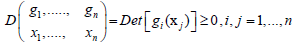for all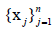such that a ≤ x1,<x2 <….<xn ≤ b, and the n-dimensional subspace G=[g1,…,gn] of C[a, b] will be called a Chebyshev subspace [1-4]. Using the continuity of the determinant, it can be shown that the sign of the determinant is constant , so we will assume that the sign of the determinant is always positive through this paper (replace g1 by -g1 if necessary). And the finite set of functions {g1,….gn} and C[a, b] is called a Weak Chebyshev system on [a, b] if it is linearly independent andfor allsuch that a ≤ x1,<x2 <….<xn ≤ b, and the n-dimensional subspace G={g1,…,gn} of C[a, b] will be called a weak Chebyshev subspace, C[a, b] is the space of all real-valued continuous functions. Extending an n-dimensional Chebyshev subspace which does not contain a constant function to an (n+1)-dimensional Chebyshev subspace containing a constant function was investigated . In what follows is the statement of the problem considered in this paper: Let G=[g1,…….gn] be a Chebyshev subspace of C[a, b] such that 1∉G and U={u0,u1,……un} be an (n+1)- dimensional subspace of C[a, b] where u0=1, ui=gi, i=1,….,n [6-8]. Our main purpose is to prove that, under certain restriction on G, U is a Chebyshev subspace of C[a, b] if and only if it is a Weak Chebyshev subspace of C[a, b]. An example illustrating that the preceding assertion is not true in general is presented and some related results are given at the end of the last section.

#### Preliminary

We start this section by the following well known theorem [3,5].

Theorem

For an n-dimensional subspace G of C[a, b], the following statements are equivalent.

(i) G is a Chebyshev subspace.

(ii) Every nontrivial function g ∈ G has at most n-1 distinct zeros in [a, b].

(iii) For all points a=t0 ≤ t1 < …. < tn-1 ≤ tn=b, there exists a function g ∈G such that

g(t)=0, t ∈ {t1,…..,tn-1}

g(t)≠ 0, t ∉ {t1,….,tn-1}

(-1)I g(t)>0, t ∈(ti-1, ti), i=1,…..,n

We need the following definitions:

Definition 1: Let U be a subspace of C[a, b], x ∈ [a, b] and f ∈U such that f(x)=0. We call x an essential zero of f with respect to U, if and only if there is a g ∈U with g(x) ≠ 0.

If no confusion arises, the term "with respect to U "will be omitted.

Definition 2: Let f ∈C[a, b] and a ≤ t1<,….,< tn ≤ b be zeros of f. we say that these zeros are separated if and only if there are s1,…..,sn-1 in [a, b] with

ti < si < ti+1

Such that

f(si) ≠ 0, i=1,……n-1.

The following theorem is a version of theorem 1 of Stockenberg .

Theorem

Let G be an n-dimensional Weak Chebyshev subspace of C[a, b]. Then the following statements hold.

1. If there is a g ∈ G with n separated, essential zeros a ≤ t1 <…< tn ≤ b, then g(t)=0 for all t with t ≤ t1 ≥ tn.

2. No g ∈ G has more than n separated, essential zeros.

#### The Main Result

We start this section with the following lemma.

Lemma

Let G={g1,…,gn} be an n –dimensional Chebyshev subspace of C[a, b] such that 1 ∉ and U={u0,u1,….,un} be an (n+1)-dimensional subspace of C[a,b] where u0=1, ui=gi, I=1,…..,n. If there are two nontrivial functions h, k∈ U and a set of n pointswith

a ≤ x1< x2< …..< xn ≤ b

such that

h(xi)=k(xi)=0, i=1,…, n,

then there is a nonzero constant λ such that h(x) – λk(x) for every x∈ [a, b].

Proof: Write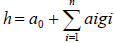, if a0=0 then h ∈ G and from theorem (1)

h(x)=0 for every x ∈[a, b], so a0 ≠ 0 then h (xi)=0,i=1,….,n, whereSimilarly, if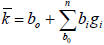, then b0 ≠ 0 and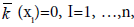where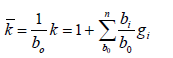Now let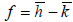, then f is an element of the n-dimensional Chebyshev subspace G with f(xi)=0, i=1,….,n, so f ≡ 0 and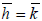, taking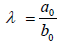, we have λ ≠ 0 and h(x)=λ k(x) for every x ∈ [a, b].

Assumption A: We say that the subspace G of C[a, b] satisfies assumption A if for each f ∈ G such that f(x)=f(y) for some x, y ∈[a, b] with x< y there is appoint z, x<z<y such v that f (z) ≠ f (x).

Lemma

Let G=(g1,…., gn) be an n-dimensional Chebyshev subspace of C[a, b] such that 1∈G and U=(u0,u1,…, un) be an (n+1)-dimensional subspace of C[a, b] where u0=1, ui=gi, i=1,…, n. If G satisfies Assumption A, then the zeros of each nontrivial function h ∈ U are separated and essential.

Proof: Let h be a nontrivial element of U such that h(x)=h(y)=0 for some x, y with a ≤ x < y ≤ b. If h ∈ G, then n ≤ 3, for otherwise h ≡ 0, and since G is an n-dimensional Chebyshev subspace of C[a, b], there is a point z ∈ (x, y) such that h(z) ≠ 0. If h∉ G, then h=α+g, where α ≠ 0 and g∈G, hence g(x)=g(y)=-α, but G satisfies Assumption A, so there is a point z∈ (x, y ) such that g(z) ≠-α that is h (z)≠ 0, this shows that the zeros of h are separated. For the second part of the assertion of the lemma, it is clear that each zero of any nontrivial element of u is an essential zero, that is because 1∈ U.

Remark 1: Note that if 1 ∉ U and 0 ∉ f ∈U, f(x)=0, then since G is a Chebyshev space, x is an essential zero for f. Indeed, there is an element g ∈G such that g(x) ≠ 0.

Theorem

Let G=(g1,… gn) be an n-dimensional Chebyshev subspace of C[a, b] such that 1 ∈ G and U=(u0, u1,….,un) be an (n+1)-dimensional subspace of C[a, b] where u0=1, ui=gi, i=1,…., n. If G satisfies Assumption A, then U is a Chebyshev subspace of C[a, b] if and only if it is a Weak Chebyshev subspace of C[a, b].

Proof: One direction is trivial.

For the other direction, suppose U=(u0, u1, ….. un) is an (n+1) – dimensional Weak Chesbyshev subspace of C [a, b] where u0=1, ui=gi, I=1, n and G=(g1,… gn) is an n-dimensional Chesbyshev subspace of C[a, b] satisfying Assumption A. Let ū be a nontrivial element of U such that we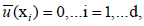a ≤ x1 <. xn+1 ≤ b,

If d>n+1, then by lemma (2) together with theorem (2) we must have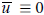, so d ≥ n+1, if d≤ n, then is nothing to prove, so to this end, we will assume that d=n+1 and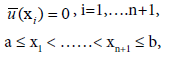again from lemma (2) and theorem (2) we must have a=x1 or xn+1=b and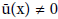for all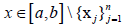Writing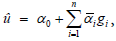then α0 ≠ 0 that is because G is an n-dimensional Chebyshev subspace of C[a, b].

Taking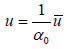, then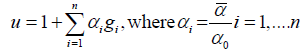u(xi)=0, I=1,…, n+1

and

u (x) ≠ 0 for all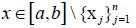The rest of the proof is divided into several cases.

Case A: a=x1 and x1+1=b.

Since G is an n-dimensional Chebyshev subspace of C [a, b], then for any point q ∈ (xn, b) there is a function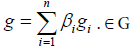such that g (yi)=1, I=1, ….., n, where yi=xi, i=1, ……..n-1 and yn=q.

Taking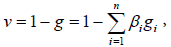Then v is a nontrivial element of U with

v (yi)=0, i=1,…..n,

a=y1 < …..,< yn < b,

and if there is a point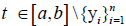such that v(t)=0, then by theorem (2) we must have t=b, hence u and v are two dimensional elements of U such that

u (xn+1)=v(xn+1)=0,

u (xi)=v(xi)=0, i=1, ….,n-1

And by lemma (1) there is a non-zero constant λ such that u=λ v, this implies that

u (ti)=0, I=1, ….n+2

Where ti=xi, i=1,….n, tn+1=yn

And tn+2=xn+1=b

This means that u has at least n+2 separated zeros in[a, b] which implies that u=v ≡ 0 contradicting the fact u and v are nontrivial elements of U, hence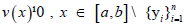. It is clear that

u(x) ≠ 0, x∈ (xn, b), u(xn)=u(b)=0

and

v (yn)=0, yn ∈ (xn, b), v(t)≠ 0 for all t ∈ [ xn, b]\{yn},

and if x ∈ [xn, yn], y ∈ (yn, b) then sign v(x)=-sign v(y), subsequently, We treat four different subcases.

Case A1: u (x) < 0 for all x ∈ (xn, b) and v(x) > 0 for all x∈ [xn, yn],

then v(x) <0 for all x ∈ (yn, b], taking w=u–v, we have

w(xn)=-v(xn) <0 and w(yn)=u(yn) >0

by the continuity of w, there is a point s ∈ (xn, yn) such that w(s)=0,

hence we have:

w(zi)=0, i=1,…..n, where zi=xi, I=1,….n-1 and zn=s.

But w belongs to the n-dimensional Chebyshev subspace G of C [a,b].

Hence w ≡ 0 and it follows that u=v and

u(ti)=0, I=1, …n +2

Where

ti=xi, I=1, …n,

tn+1=yn and tn+2=xn+1=b

So u must be identically zero.

Case A2: u(x) > 0 for all x ∈ (xn, b) and v(x) < b0 for all x ∈ [xn, yn], then v(x) <0 for all x∈ [xn,yn],

then v(x) > 0 for all x∈ (yn, b], again taking w=u-v, we have

w(yn)=u(yn) >0 w(b)=-v (b)<0,

and there is a point s ∈ (yn, b], such that w(s)=0, so w has at least n distinct zeros in [a,b]. A similar argument as in case A1 shows that u must be identically zero.

Case A3: u(x)< 0 for all x ∈ (xn, b) and v(x)<0 for all x ∈ [xn, yn),

then v(x) > 0 for all x∈ (yn, b], taking w=u-v, we have

w(yn)=-v(xn) > 0 and w (yn)=u (yn)< 0,

and continuing exactly as in case A1, we conclude that u must be identically zero.

Case A4: u(x) < 0 for all x ∈ (xn, b) and v(x) > 0 for all x∈ [xn, yn),

then v(x) <0 for all x∈ (yn,b], taking w=u-v, we have

w (yn)=u (yn) < 0 and w( b)=-v (b) >0 and an argument similar to that of case A2 shows be identically zero.

Case B: a< x1 and xn+1=b

As in case A, for any q ∈ (xn, b) there is a function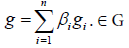Such that g(yi)=1, I=1,…….,n, where

yi=xi, i=1,…n-1 and yn=q

Taking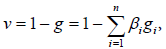Then v is a nontrivial element of U with

v(yi)=0, I=1, ….n,

a< y1< ……< yn< b

and if there is a point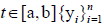such that v(t)=0,then by theorem (2) we must have t=b or t=a.

If t=b, then u and v are two nontrivial elements of U such that

U(xn+ 1)=v(xn+1)=0,

u(xi)=v(xi)=0, I=1, …n-1

and by lemma 1 there is a nonzero constant λ such that u=λ v, this implies that

u(ti)=0, i=1,….n+ 2

where ti=xi, i=1, …..n,

tn+ 1=yn

and tn+2=xn+1=b

so u has at least n+2 separated zeros in[a, b] which implies that u=v ≡ 0 and this is a contradiction.

so t ≠ b and the situation becomes exactly as in case A, proceedings as in case A we conclude that u must be identically zero.

Case C: a=x1 and xn+1< b

The proof of this case requires that n ≥ 2 and the proof for n=1 will be given in remark (2).

Now, for any point p ∈ (a,x1) there is a function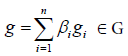such that

G(yi)=1, i=1,…., n,

Where y1=p and yi-1=xi, i=3,…n+1.

Taking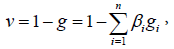Then v is a nontrivial element of U with

v(yi)=0, i=1,….,n,

a < y1 < ….. < yn < b,

and if there is a point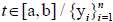such that v(t)=0, then by therome (2) we must have t=a or t=b.

If t=a, then u and v are two nontrivial elements of U such that

U(x1)=v(x1)=0, u(xi)=0, i=3,….,n+1.

A similar argument to that of the other cases leads to a contradiction.

So t ≠ a and on the interval [a, x2] we have

u(a)=u(x2)=0, u(x)≠ 0, x ∈ (a,x2)

and

v(y1)=0, y1 ∈ (a,x2),v(t) ≠ 0 for every t ∈ [a,x2]\{y1}.

If x ∈ [a,y1), y ∈ (y1,x2] then sign v(x)=-sign v(y), and as in the other cases we are presented with four different subcases. In each case, a similar argument to that of the cases in A can be used to show that the function u-v in G has at least n zeros which leads to the conclusion that u must be identically zero. Hence U is a Chebyshev subspace of C [a,b].

Remark 2: The following is the proof for theorem (3) when n=1 which is somehow more direct:

Suppose g is a non constant continuous function on [a,b] such that G=[g] is a Chebyshev subspace of C[a,b] of dimension 1 satisfying Assumption A and U=[1,g] is a subspace of C[a,b] of dimension 2. If U is not a Chebyshev subspace, then there is a nontrivial element u=α +βg of U such that u(x1)=u(x2)=0 where a ≤ x1 < x2 ≤b, clearly α ≠ 0 and β ≠ 0, so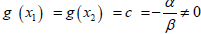.

By lemma (2) there is a point y1, x1< y1< x2 such that g(y1)=d≠ c.Taking x1= z1, y1=z2 and x2=z3,then

az1 < z2 < z3b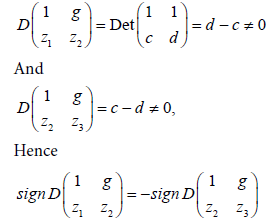This shows that U is not a weak Chebyshev subspace and the theorem is proved.

The followings example illustrates that theorem (3) is not true in general is proved.

Example 1

Let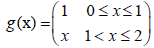G={g} is a Chebyshev Subspace of C[0,2] of dimension 1, if U=(1,g) and ≤ x1 < x2 ≤ 2, then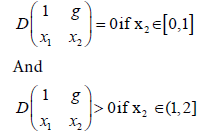That is U is a 2-dimensional weak Chebyshev Subspace of C[0,2] but not a Chebyshev Subspace.

If H is n-dimensional subspace of C[a, b], then it is possible that H is a Chebyshev subspace on one of the intervals (a, b] or [a, b) but not on the closed interval [a,b] as illustrated in the following example.

Example 2

Let H=(sin x, cos x), it can be easily checked that H is a Chebyshev subspace of dimension 2 on each of the intervals (0,π] of dimension 2.

In next result we give a necessary and sufficient condition for an ndimensional Chebyshev H on (a,b] or [a,b) to be a chebyshev subspace on the closed interval [a,b].

Theorem

Let H be an n-dimensional subspace of C [a, b] such that H is a Chebyshev subspace on (a,b] or on [a,b), then H is a Chebyshev subspace on [a, b] if and only if each function hi, I=1,…n can have at most n-1 distinct zeros on [a,b] whenever H=[h1,…hn].

Proof: If (h1,…hn) is a basis of H such that for some s ∈ {1,…n},hs has at least n zeros on [a, b],then clearly H is not a Chebyshev Subspace on [a,b].For the other direction, suppose H is Chebyshev subspace on.

I=(a, b] but not on [a, b], there is a non-trivial element u ∈ U such that

u(z1)≠ 0, u (zi)=0, i=2, …n.

Since H is a Chebyshev subspace on (a, b], there is a subset E={h1,…. hn} and H such that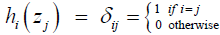The elements of E are linearly independent and H={h1,….h2},write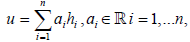Then

0 ≠ u (z1)=a1h1(z1)=a1,

0=u (zi)=aihi(zi)=ai, I=2, ……n,

Hence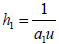which has n zeros on [a, b] and this is a contradiction.

Using a similar argument when I=[a, b) leads to a contradiction and the theorem is proved.

#### References

Select your language of interest to view the total content in your interested language

### Article Usage

• Total views: 970
• [From(publication date):
July-2017 - Dec 08, 2019]
• Breakdown by view type
• HTML page views : 884Can't read the image? click here to refresh